# How Area Formulas of Triangles, Parallelograms & Trapezoids Relate

Lesson Transcript
Instructor: Laura Pennington

Laura received her Master's degree in Pure Mathematics from Michigan State University, and her Bachelor's degree in Mathematics from Grand Valley State University. She has 20 years of experience teaching collegiate mathematics at various institutions.

Triangles, parallelograms, and trapezoids are all two-dimensional shapes that show up in the world around us. In this lesson, we'll look at the areas of these shapes and how they relate to each other.

## Three Different Shapes

Let's talk about shapes, three in particular! They are the triangle, the parallelogram, and the trapezoid. These three shapes are related in many ways, including their area formulas. The area of a two-dimensional shape is the amount of space inside that shape. Before we get to those relationships, let's take a moment to define each of these shapes and their area formulas.

Let's first look at parallelograms. A parallelogram is a four-sided, two-dimensional shape in which opposite sides are parallel and have equal length.

To find the area of a parallelogram, we simply multiply the base times the height.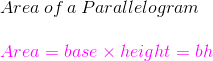Now, let's look at triangles. You've probably heard of a triangle. A triangle is a two-dimensional shape with three sides and three angles.

To find the area of a triangle, we take one half of its base multiplied by its height.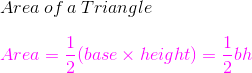Finally, let's look at trapezoids. A trapezoid is lesser known than a triangle, but still a common shape. A trapezoid is a four-sided, two-dimensional shape with two parallel sides. Trapezoids have two bases. Those are the sides that are parallel.

To find the area of a trapezoid, we multiply one half times the sum of the bases times the height.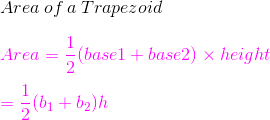An error occurred trying to load this video.

Try refreshing the page, or contact customer support.

Coming up next: Relationships Among Area Formulas of Various Quadrilaterals

### You're on a roll. Keep up the good work!

Replay
Your next lesson will play in 10 seconds
• 0:04 Three Different Shapes
• 1:12 Area Relationship
• 3:07 Lesson Summary
Save Save

Want to watch this again later?

Timeline
Autoplay
Autoplay
Speed Speed

## Area Relationship

Alright! Now that we got all the definitions and formulas out of the way, let's look at how these three shapes' areas are related. To get started, let me ask you: do you like puzzles? That probably sounds odd, but as it turns out, we can create parallelograms using triangles or trapezoids as puzzle pieces. This fact will help us to illustrate the relationship between these shapes' areas.

Let's first look at the relationship between parallelograms and triangles. First, let's consider triangles and parallelograms. Notice that if we cut a parallelogram diagonally to divide it in half, we form two triangles, with the same base and height as the parallelogram.

We see that each triangle takes up precisely one half of the parallelogram. From this, we see that the area of a triangle is one half the area of a parallelogram, or the area of a parallelogram is two times the area of a triangle. By looking at a parallelogram as a puzzle put together by two equal triangle pieces, we have the relationship between the areas of these two shapes, like you can see in all these equations.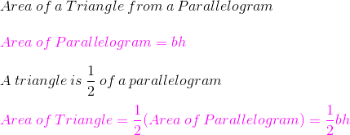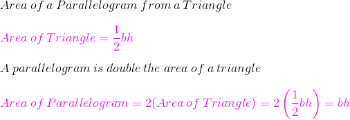To unlock this lesson you must be a Study.com Member.

### Register to view this lesson

Are you a student or a teacher?